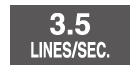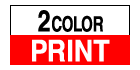# DR-140TM

Close

• The colors may differ slightly from the original.

## DR-140TM

Past Model

Dest-Top Type
14 digits
3.5 line-per-second printing
The value indicates the number of lines printed per second.
2-color printing
Positive values are shown in black, and negative values are shown in red for easy checking.

## Features

### Cost, Selling Price and Margin Calculations

Perform the operations shown to the right to calculate cost, selling price, and margin.

### Tax Calculations

Set the rate you want for easy calculation of amount plus tax, amount less tax, and tax amount.

## Printing Sample

### Cost

What is the cost of an item that sells for \$150 after a 30% margin is added?

### Selling Price

What would the selling price be for an item that costs \$120 after a 40% margin is added?

### Margin

What is the margin on an item that costs \$1,000 and sells for \$2,000?

• Print sample: DR-120TM

## Specifications

•Dest-Top Type

•14 digits

•3.5 line-per-second printing The value indicates the number of lines printed per second.

•2-color printing Positive values are shown in black, and negative values are shown in red for easy checking.

•Regular percent Regular percentage calculations.

•Line printing Line printing for higher speed, superior print quality, and quieter operation.

•Tax calculation Automatic calculation of price plus tax, price less tax, discount, selling price, tax amount, discount amount, and margin amount.

•Cost/Sell/Margin Calculate the cost, selling price, or margin of profit on an item, given the other two values.

•Mark-up/Mark-down All the mark-up/mark-down capabilities of an adding machine for simplified cost and profit calculations.

• Rounding selector F: Floating decimals. CUT: Values are cut off. UP: Values are rounded up. 5/4: Values are rounded off.

• Decimal selector 4,3,2,1,0 : Specifies the number of decimal places as 4,3,2,1,0. ADD2: 2 decimal places are assumed for entries and results of all addition and subtraction operations.

•Key rollover Key operations are stored in a buffer, so nothing is lost even during high-speed input.

••Plastic keys Designed and engineered for easy operation.

### Size of case / Total weight

• Dimensions (D × W × H) : 382×214.5×109.3mm
• Weight : 1700g